## Profit and Loss For SBI PO Set – 4

1)  21 articles were bought for Rs. 6531 and sold for Rs. 9954. How much was the approximate profit percentage per article?

a) 56%

b) 43%

c) 52%

d) 49%

e) 61%

c) 52%

Explanation

Cost price of 21 things=Rs.6531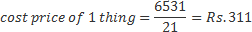Selling price of 21 thing=Rs.9954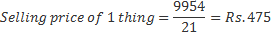Profit on 1 thing= (475-311)=Rs.163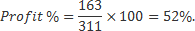2) The cost price of an article is Rs. 480. If it is to be sold at a profit of 6.25 percent, how much would be its selling price?

a) Rs. 510

b) Rs. 530

c) Rs. 503

d) Rs. 519

e) None of these

a) Rs. 510

Explanation

Cost price=480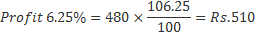3) The cost of 3 digital cameras and 5 cell phones is Rs. 35,290. What is the cost of 9 digital cameras and 15 cell phones?

a) Rs. 1,05,870

b) Rs. 8,46,960

c) Rs. 1,76,450

d) Cannot be determined

e) None of these

a) Rs. 1,05,870

Explanation

Cost of (3 digital cameras+5 cell phones)=Rs.35290

Cost of (9 digital camera+15 cell phones)=3×(3 digital camera +5 cell phones)

=3×35290

=Rs.105870.

4) 17 articles were bought for Rs. 3,910 and sold for Rs. 4,590. How much was the approximate profit percentage per article?

a) 17%

b) 12%

c) 9%

d) 21%

e) 25%

Explanation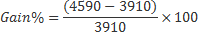=Rs.17%

5) The owner of a computer shop charges his customer 13% more than the cost price. If a customer paid Rs. 15,933 for a computer, then what was the cost price of the computer?

a) Rs. 14,100

b) Rs. 16,500

c) Rs. 12,700

d) Rs. 18,200

e) None of these

Explanation

Let cost price of the computer is x. Then
x+13x/100

= 15933

113x = 15933×100 = 1593300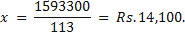6) The owner of an electronics shop charges his customer 22% more than the cost price. If a customer paid Rs. 10,980 for a DVD player, then what was the cost price of the DVD player?

a) Rs. 8,000

b) Rs. 8,800

c) Rs. 9,500

d) Rs. 9,200

e)

Explanation

Selling price=Rs.10980

C.P+22% C.P=10980

122%=10980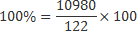=Rs.9000

7) Savita sold an item for Rs. 6,500 and incurring a loss of 20%. At what price should she have sold the item to have gained a profit of 20%?

a) Rs. 10,375

b) Rs. 9,750

c) Rs. 8,125

d) Cannot be determined

e) None of these

Explanation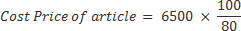= Rs. 8125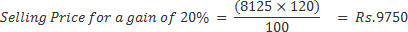8) A shopkeeper sold some articles at the rate of Rs. 35 per article and earned a profit of 40%. At what price each article should have been sold so that 60% profit was earned?

a) Rs. 45

b) Rs. 42

c) Rs. 39

d) Rs. 40

e) None of these

Explanation

Selling price=140% of cost price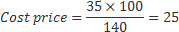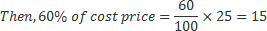To set a profit of 60% the selling price=15+25=Rs.40

9) A shopkeeper purchased 200 bulbs for Rs. 10 each. However, 5 bulbs were fused and had to be thrown away. The remaining were sold at Rs. 12 each. What will be the percentage profit?

a) 25

b) 15

c) 13

d) 17

e) None of these

Explanation

200 bulbs at Rs.10=2000

5 bulbs defective

195 bulbs sold at 12

Selling price=195×12=2340

Profit=2340-2000=340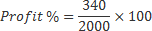10) Ranjeet purchased an item for Rs. 3,500 and sold it at the loss of 25%. From that amount he purchased another item and sold it at the gain of 20%. What is his overall gain/loss?

a) Loss of Rs. 240

b) Gain of Rs. 120

c) Loss of Rs. 350

d) Neither gain nor loss

e) None of these

c) Loss of Rs. 350

Explanation

1st Selling price=Rs.3500-25% 3500

=3500-875=Rs.2625

2nd Selling price=2625+26%2625

=2625+525=Rs.3150

Loss=3500-3150

=Rs.350.